# Vintage Tube Radio Restoration

Feb 17, 2016## Introduction

This article describes the restoration of a vintage American 1946 Mantola R654-PM superheterodyne AM tube radio receiver to original working order. A similar paper by P. Hiscocks of Syscomp Electronic Design: Measurements On A Tube Radio (2011) provides measurements and theory for an RCA version of the classic All American Five (AA5) radio, similar to the Mantola model discussed here. The schematic diagram for the RCA version is shown at the end of this article with the corresponding tubes for the Mantola model indicated. Bret Menassa provides a good overview of radio alignment (2009), the procedure for adjusting the tuned circuits of a receiver to obtain maximum performance, as well as providing basic terms and illustrating with several examples of vintage AM receivers.

## Inspection and Testing

The circuit schematic diagram for the Mantola R654-PM is available, along with detailed circuit information, component part numbers and voltage/resistance measurements, from radiomuseum.org. Initial visual inspection indicated that the power line-chord was frayed and extremely brittle, requiring replacement. The chassis was removed from the cabinet and the circuit wiring, circuit components and tubes were visually inspected. No sign of unusual wear or failure (burn marks etc.) was observed and all solder joints looked shiny. The wiring in these types of receivers was typically point-to-point as in this set. The chassis, tubes and other top-mounted components were cleaned. Tubes were removed and all contacts were sprayed with contact cleaner and the tubes reinstalled. Components and wires were moved slightly to check for hidden brittle connections. The five octal-base standard size tubes are shown below along with their locations and functions. Three of the original tubes were manufactured in Canada (Marconi, RCA, Addison):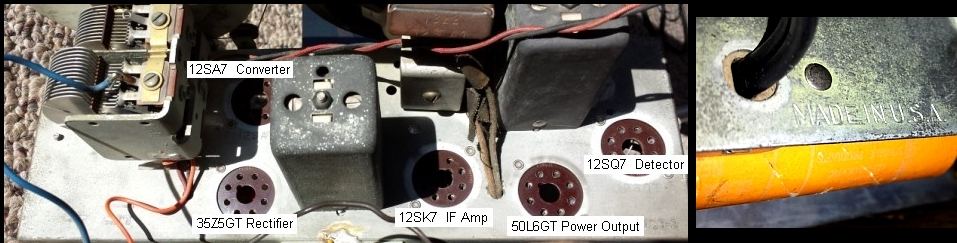The original unpolarized line-chord was unsoldered after removing the unusual cam-lever chord-lock mechanism shown below. In this older radio design, without power transformer isolation from the AC mains and with an unpolarized power plug, there is a safety risk even though the B- supply is electrically isolated from the chassis via a 0.1 uF capacitor in parallel with a 150 kΩ resistor. The safest modification is to use a polarized power plug with the hot side of the power cable connected to the rectifier tube, the neutral power connection to the B- connection (closest to the chassis ground connection; see schematic diagram below) and the power switch on the hot size of the line voltage. This will also allow for safe voltage measurements using the radio chassis as a ground connection to a normal power-line grounded oscilloscope. Nevertheless, as an important added safety test, simple AC and DC voltage measurements should be made with a floating DVM between the radio chassis and the ground connection of a scope, ensuring there is no voltage difference, before making a grounded scope connection. These measurements should be performed with the radio power switch both OFF and ON. (NOTE: if the power switch is wired on the NEUTRAL side of the power line cable, with the power switch off, there will likely be a shock hazard with a high line voltage present between the radio chassis and an external power-line grounded test instrument. For example, in the example RCA AA5 schematic below, and with the polarized connection discussed, the power switch should be changed to the HOT side of the circuit). A new line-chord was stripped to suitable length and soldered to the required locations to route the hot and neutral sides of the power cable as discussed:The radio was then powered up. The #47 pilot lamp lit up briefly and then went dark and this behaviour repeated with each power-up sequence. This suggested an intermittent contact in the circuit, possibly in a tube filament heater. In this type of transformerless AC/DC receiver, the tube filaments are connected in series, with the total voltage drop across all series-connected filaments being equal to the 117 VAC line voltage and the nominal filament current draw being 150mA. If any of the 5 tube filaments fails, none of the tubes will operate including the pilot lamp! Without a tube-tester, it is still possible to check tube-filament operation. Therefore each of the 5 tubes was removed and tested using a dual 15V DC power supply capable of delivering the required nominal 150mA filament current (DC or AC). For the 35Z5GT rectifier and the 50L6GT output power tube, the filament was tested at 30VDC which is sufficient for this test, as shown below for the 50L6GT:The 12SQ7 detector tube was identified as having a faulty filament circuit with a momentarily intermittent filament connection. To ensure this was the problem and without having a replacement 12SQ7 tube, the 12SQ7 was removed and a 100 ohm dummy power-resistor, roughly equivalent to the filament resistance for this tube, was used across the tube-socket filament-heater terminals. This test verified that all other tubes now received power. A simple probe test using an insulated screwdriver at the grid of the 50L6GT output tube produced a buzz at the loudspeaker as expected:A replacement 12SQ7 tube along with some of the other tubes were ordered from TheTubeStore. Substitution of the faulty 12SQ7 with the new tube resulted in apparent proper operation of the receiver. However, another problem appeared when the volume control was turned up. After a moderate volume level was achieved, further increase of the volume control resulted in REDUCED and distorted output speaker volume. The circuit schematic was examined and another detailed inspection of the wiring was conducted revealing the source of the problem. The 10 MΩ grid resistor for the 12SQ7 detector tube (R7 in the similar circuit below) was disconnected from the B- supply pin at that tube. The resistor was resoldered and several other joints were resoldered. This fixed the volume control problem and operation was normal. The 150Ω power tube cathode resistor was replaced as it had drifted to 200Ω. In addition, the 0.01µF grid-coupling capacitor for the output power tube was replaced. The remaining original "good" tubes were also substituted with new NOS replacement tubes in the circuit with no noticeable change in performance indicating that the original remaining tubes were sufficiently good for normal operation. The total power consumption of the receiver was measured to be 28 W with about 20W consumed by tube filament heaters, 4W by the power tube, and the remaining power dissipated mainly by the remaining 3 tubes. Next, the receiver was aligned using a modulated 455kHz signal as described in the product specification sheets. More detailed discussions of alignment are provided by Hiscocks, 2011 and Menassa 2009. The IF transformer capacitors and the ganged tuning capacitor antenna and oscillator trimmers were adjusted for maximum signal.

Although the hum level was fairly low, an additional electrolytic filter cap was added after the filter choke with negligible improvement in receiver hum. Except for the power tube grid capacitor, none of the other capacitors were replaced and the receiver gain was impressively high with no sign of deterioration even at maximum volume (except for the effects of the AGC circuit). The audio quality from this receiver with the original 4.5" diameter loudspeaker was quite good although the speaker cone was quite brittle and a few points needed to be carefully patched with epoxy. Finally, the tuning-dial string needed replacement which was greatly facilitated by the instructions included with the schematic mentioned above: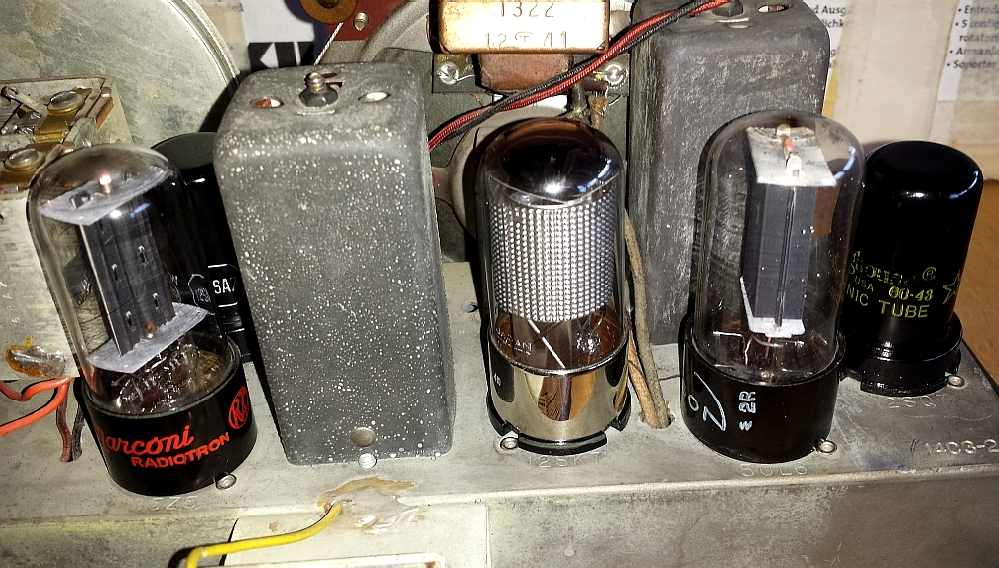## Oscilloscope Measurements

Oscilloscope measurements were taken using a 10MHz Digilent Analog Discovery USB oscilloscope. After verifying with a floating DVM that there was no AC or DC voltage between the radio chassis and the ground connection of the oscilloscope, an HP6351R 10x 10MΩ scope probe was used with the ground connected to the radio chassis for higher plate voltage measurements. For lower voltage measurements, a 1x 1MΩ probe was used in cases where scope loading was insignificant. Scope inputs were set at DC coupled for all measurements shown. The zero voltage baselines are shown by the C1 and C2 arrow markers. Voltage measurements are relative to the chassis ground, not the B- common side of the supply DC voltage. However, with a 10MΩ probe impedance, the impedance of the 0.1 uF capacitor in parallel with a 150 kΩ resistor (components C4 and R1 in schematic below) is negligible at frequencies from 60 Hz and above so voltage measurements are sufficiently accurate. Vertical and horizontal ranges vary depending on the measurements: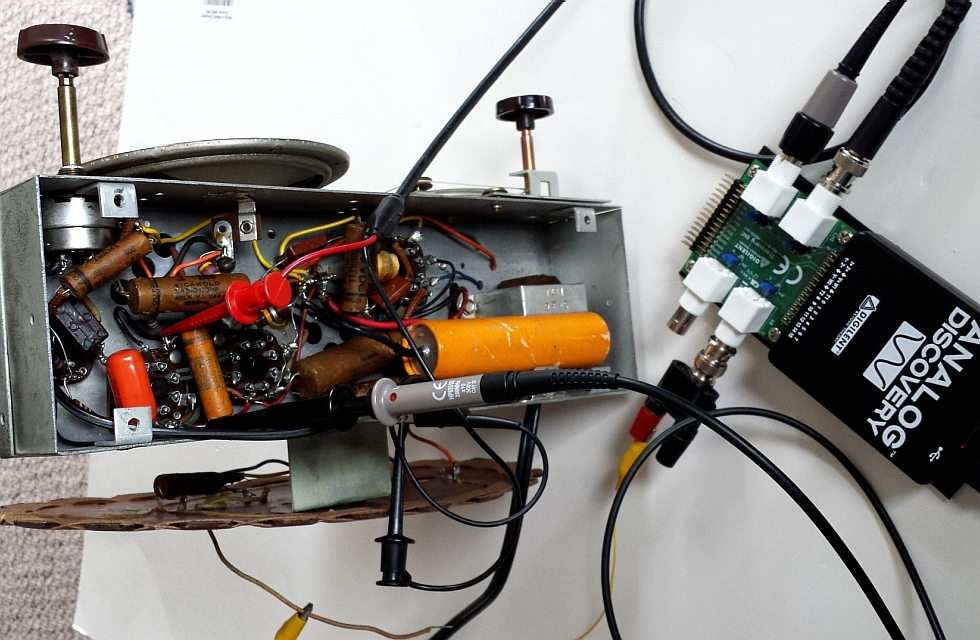### Power Supply

Earlier versions of this Mantola radio, such as the one tested here, used a choke CLC filter in the rectifier stage compared to many other AA5 sets that used simple C filtering (as in RCA example below) or CRC filtering: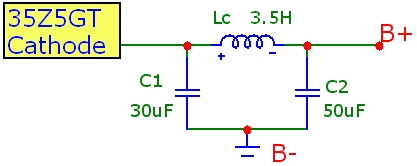The traces below show the power supply voltages at the 35Z5GT rectifier tube and the filtered B+ voltage after the LC filter using the 10X probe: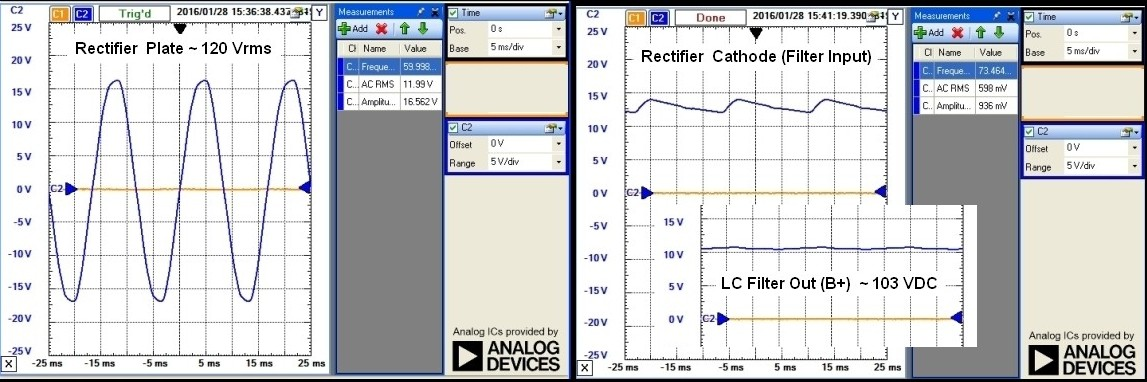### IF Alignment

The traces below show an audio-modulated IF signal at the output of the 2nd IF transformer (input to the 12SQ7 detector tube) using the 10x probe. The 600Hz modulated 920kHz RF carrier was injected at the antenna input (grid of converter tube). The upper trace clearly shows the audio modulated IF carrier, while the lower expanded trace shows the ~ 455kHz IF carrier: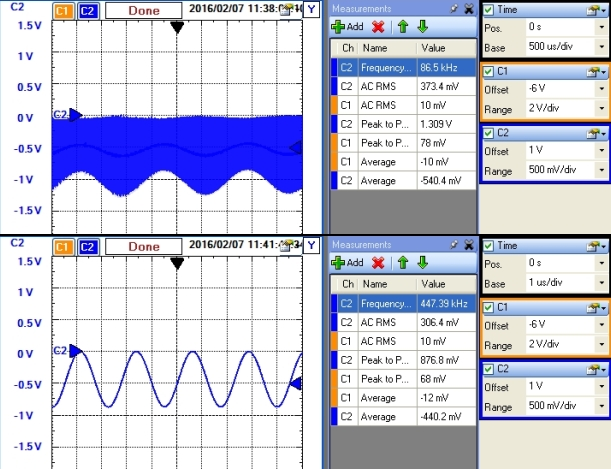The Bode plot below shows the tuned IF stage response. Using a Digilent Analog Discovery Function Generator, an RFsignal was swept over a range centered around the nominal IF frequency of 455kHz. This signal was applied to the grid of the first (converter) tube, and the response was measured at the output of the 2nd IF transformer tank at the input of the 12SQ7 detector. The results below show good alignment to 455kHz to within 0.5kHz: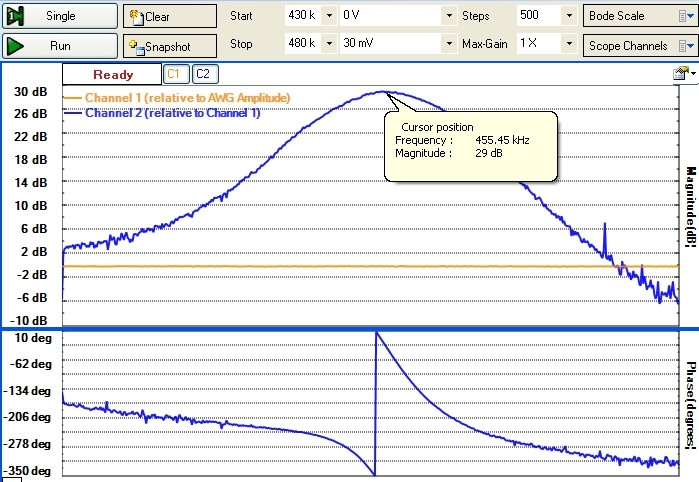### Local Oscillator

Probing the local oscillator (LO) signal at the pin 5 oscillator-grid of the 12SA7 pentagrid converter tube is shown below. The scope traces show the variation of the LO frequency from the minimum (~ 540kHz) and maximum (~ 1630kHz) tuned frequencies. The LO voltage amplitude is 11 - 13Vpeak: The LO frequency measured this way will be modified somewhat by the probe capacitance:Most receivers of this type use high-side injection in the mixer with the LO frequency being higher than the desired RF frequency being tuned it. In this case, with an IF=455kHz, the LO frequency should be 455kHz higher than the tuned RF frequency. Since the scope probe above introduces about 16pF of capacitance to the oscillator circuit, the LO frequency will be pulled to a lower value. For example, at the 1630 kHz high frequency end of the standard AM reception range, the frequency measured above is 1913kHz, substantially lower than the expected value of 455+1630 = 2085kHz. At the high-frequency end of the AM band, where the oscillator ganged capacitor rotor fully open with a low capacitance ~ 10 - 20pF, the relative effect of scope probe capacitance on the LO frequency is large. To measure the LO oscillator frequency more accurately, a Radio Shack DX-440 digital short-wave receiver was used with antenna in close proximity to the oscillator circuit of the radio being tested. The LO frequency results, 970 and 2100 kHz, are shown below and are close to the expected values (the low frequency end of the tuning range was determined separately to be 520kHz, somewhat lower than the standard 540kHz value):### Output Transformer

The DC resistance of the audio output transformer primary winding was measured to be 270Ω (compared to the spec. of 150Ω). The ratio of the primary to secondary AC audio voltage is the transformer turns ratio Np/Ns. This voltage ratio was measured two different ways: (a) with the radio powered off, the output power tube removed and a 1kHz signal directly driving the primary of the output transformer and (b) with a 1kHz modulated 1MHz carrier frequency with the radio powered up and voltages measured again on the primary and secondary sides of the output transformer. The results are identical with Np/Ns=44 as shown below for the first measurement with a X1 probe. With the transformer secondary connected to a speaker impedance of say Zs=4Ω, the secondary load impedance is transformed to a primary load impedance (i.e. the load impedance seen by the output power tube) as the square of the turns ratio: Zp=(Np/Ns)^2*Zs = 7.7kΩ (compared to the spec. of a primary impedance of 2.3kΩ with a secondary speaker impedance of 3.15Ω). The primary load impedance was also directly measured using method (a) and with a 1kΩ resistance in series with the transformer primary and comparing the voltages across the resistor and transformer primary. This yielded a primary impedance of 4.7kΩ Using the measured turns ratio of 44, this would imply a secondary speaker impedance of 2.4Ω, fairly close to the speaker specification of 3.2Ω.### Power Tube

The dual traces below show the plate voltage (upper Ch2 traces in all images with 10x probe) and the control grid, cathode and transformer output voltages for a 600Hz audio signal at the 50L6GT power tube stage. All lower (Ch 1) traces are with the x1 probe. The DC plate, cathode and control grid voltages, measured without an audio signal using a 10MΩ DVM, were 93, 6 and 0 VDC respectively. Therefore, with a 150Ω cathode bias resistor, the DC plate current was 40mA and the plate dissipation was 3.5W, well below the maximum 10W plate dissipation for this power tube. (Since this is a pentode, the cathode current actually consists of both the plate and screen-grid currents. The true plate current was determined by measuring the DC resistance of the output transformer primary of 270Ω and measuring the DC voltage drop across the primary of 10.2VDC giving Ip=38mA, close to the 40mA cathode current. The screen-grid current was therefore 2mA). From the measured AC plate and cathode voltages (and hence AC plate current), the load impedance of the transformer primary at 600Hz was determined to be 3.3kΩ which is somewhat different than the value determined above. Note that the plate and cathode voltages (and hence plate current) are almost exactly 180° out of phase demonstrating that the transformer (with a speaker secondary load) behaves as an ideal resistive plate load at the 600Hz test frequency. From the AC grid voltage measurement, the AC voltage gain is ~ 10x. The unbypassed 150Ω cathode resistor reduces the gain substantially but lowers distortion through regenerative feedback (see details below for bypassed cathode resistor). The expected AC gain a for a pentode amplifier with an unbypassed cathode resistor is (Brophy 1966):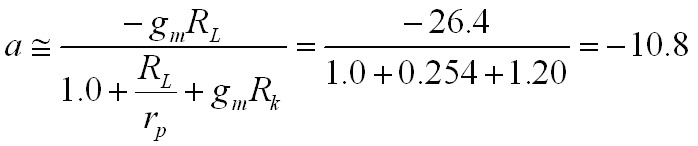where gm is the tube transconductance = 0.008mhos, RL is the load impedance = 3.3kΩ, rp is the plate resistance =13kΩ and Rk is the cathode bias resistor = 150Ω. Values for the transconductance and plate resistance are representative values for Vcc=110V from the 50L6GT data sheet. The gain result agrees quite well with the measured gain between 600 Hz to 1kHz (see below). Note that if the cathode resistor were bypassed with say a 50uF capacitor essentially shorting out the cathode resistor over the main audio spectrum, the audio gain would be doubled to ~ 21. The ratio of the measured transformer output to plate AC voltages is ~ 44 which is the transformer turns ratio Np/Ns in agreement with the results above: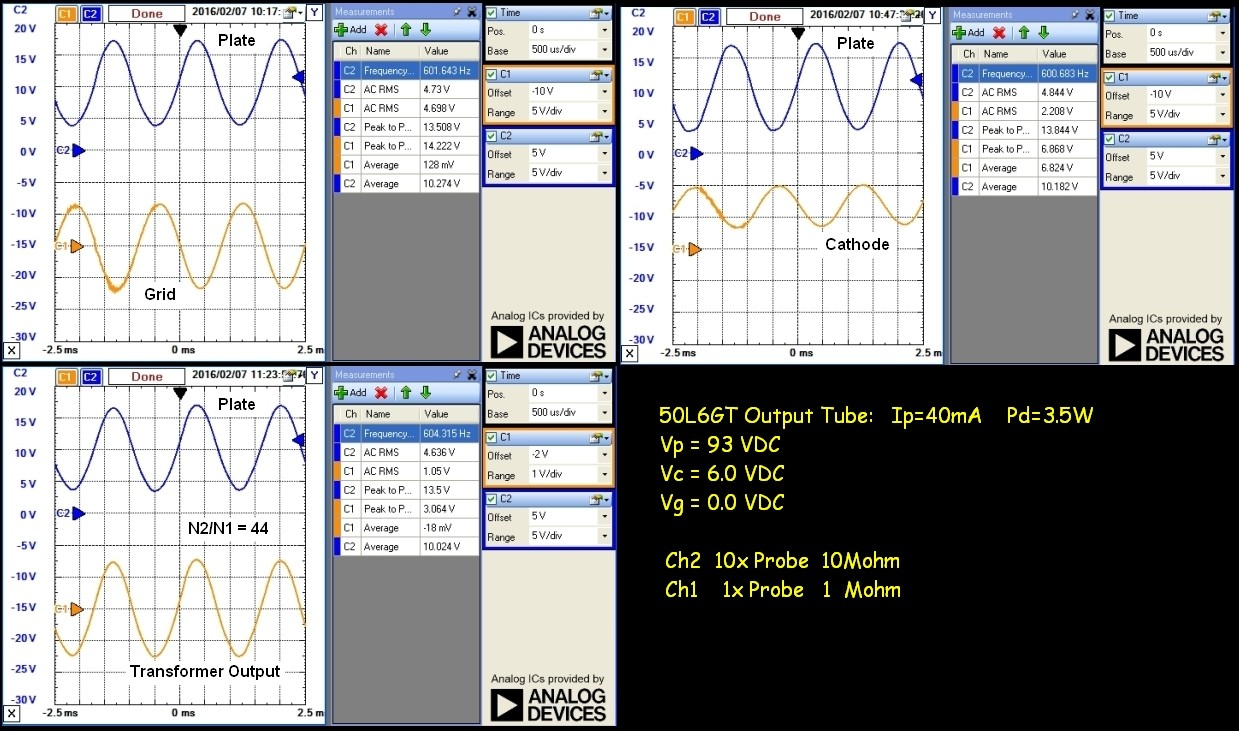The undistorted available audio output power (into the transformer primary load) can be understood by examining the dashed red load line shown below. The quiescent plate voltage (voltage between plate and cathode) is about 85VDC (since the cathode voltage is ~ 6V). The characteristics shown correspond to a screen-grid voltage Ec2 of 110VDC compared to 100VDC for this radio. This lower screen grid voltage lowers the set of curves so the actual equivalent control-grid bias is about -8V for a quiescent plate current of ~ 40mA as shown. The slope of the load line is the load impedance ~ 3.3kΩ:If the plate voltage swing at the lower voltage (increasing control grid voltage) reaches the "knee" of the characteristic curves at ~ 30V along the load line, distortion due to clipping will result. The maximum power output before excessive distortion occurs is approximately the product of the rms plate voltage and current swings up to this clipping limit:The graph and measured response indicate a maximum output power of ~ 0.75W. Reducing the load resistance to ~ 2kΩ will increase the output power to just over 1W.

### Audio Frequency Response

The audio frequency response of the output power tube stage from 50Hz to 20kHz is shown below as a bode plot. The 1Vpeak reference audio signal was applied to the base of the 50L6GT tube, the frequency swept, and the response measured across the primary side of the output transformer. As measured above, the audio gain is about 10 at a frequency of 600 Hz. Since a 10X probe was used in this mesurement, the gain relative to the input signal is about 0dB although the gain varies considerably over the audio spectrum. The -3db response extends from about 500Hz to 4.5kHz. There is a resonance in the response around 200Hz. For an ideal output transformer with an ideal resistive speaker load, the gain would be much flatter with frequency. However, the speaker load is far from resistive and varies considerably with frequency. This change in impedance is reflected to the primary of the transformer and the transformer itself is non-ideal and therefore doesn't exactly reflect the speaker impedance to the primary as the turns-ratio squared. This results in the rising gain up to ~ 2.5kHz. A 0.2uF capacitor across the transformer primary is used to reduce the possibility of higher frequency oscillations in the plate circuit and in combination with the nominal load impedance (3.3kΩ) causes the high-frequency gain rolloff above 3kHz. A passive measurement of the frequency response of the output transformer and speaker alone (power off and output power tube removed) indeed shows the impedance of the transformer+speaker increasing continuously to over 8kHz at a rate consistent with the slope shown before the 0.2uF shunt capacitance becomes effective: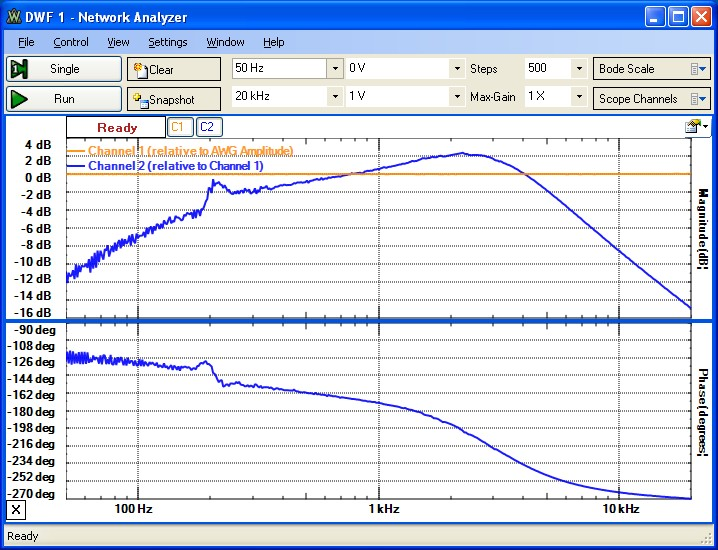## Summary

This radio restoration demonstrates that it is possible to return an AM tube radio receiver of 70 years to useful service by basic testing and trouble-shooting procedures, without the requirement of a tube tester. For the 70 year-old set tested here, it was only necessary to replace the power chord, one faulty tube, two resistors and to resolder a few connections. It was not necessary to replace any capacitors or other components. The safely guidelines discussed above are a critically important consideration when testing these types of All American 5 radio sets without power transformer isolation.

## Power Tube Gain

The schematic diagram below shows a typical single-ended power output stage with output transformer load and a bypassed cathode-bias resistor Rk. The screen-grid for the Mantola radio is connected directly to Vb (no protective screen-grid resistor!). The parallel impedance of Rk and Ck is Zk. A shunt capacitive impedance Zs across the transformer primary helps to prevent oscillations and for small-signal analysis acts essentially in parallel to ZL and thus rolls off the high-frequency gain. The component values and voltages are similar to the values for the radio discussed above except for the added Ck. The load impedance ZL for the plate circuit is approximately the output speaker impedance reflected to the transformer primary via the turns ratio ZL ~ Zsp*(Np/Ns)^2. For initial load-line analysis and gain calculations, Zsp and therefore ZL are approximated as fixed resistances with the speaker resistance being the nominal stated value (typically 2, 4 or 8Ω). However since speaker impedance varies with frequency and transformers are non-ideal, ZL is in general reactive and frequency dependent. :Standard small-signal gain analysis (Brophy, 1966) provides the voltage gain:where μ is the tube amplification factor, rp is the plate resistance (~ 13kΩ) and gm is the mutual conductance (~ 0.008 mhos), all evaluated at a plate voltage of ~ 110VDC from the data sheet. (ZL in this expression is the parallel combination of ZL and Zs in the schematic diagram above). If the cathode resistor is NOT bypassed, as is the case for the radio discussed here, Zk = Rk and is frequency independent. If the cathode resistor were bypassed with a typical 50uF capacitor, Zk ~ 0 over the main audio spectrum. With ZL ~ RL ~ 3.3k&Omega, the nominal mid-band gain is ~ 21. For this bypassed case, and since ZL~ Rp < rp, an approximate value for the mid-band gain is a ~ -gm*RL = 26. With a shunt capacitance across the transformer primary, the gain will roll off with at a high-frequency corner fc ~ 1/(2*π*RL*Cs). With RL ~ 3.3kΩ and Cs = 0.02uF; fc ~ 2.4kHz. Without cathode bypassing, Zk=Rk, and with ZL ~ RL, this expression reduces to that given above and the nominal gain is reduced by ~ 1/2 and the gain rolls off again at approximately fc. The frequency dependent impedance of Zsp will result in the non-uniform measured gain spectrum shown above.
[Note: The transformer bypass capacitor Zs for the Mantola radio is actually between the plate and cathode. This has the effect of extending fc to ~ 5.5kHz].

## Measuring Output Transformer/Speaker Impedance

The impedance of the combined output transformer with a speaker load can be measured as follows. With the receiver powered OFF, remove the output power tube. For the 50L6GT power tube, with the input voltage v2 applied between the cathode (pin 8) and the anode connection (pin 3), measure the AC voltage amplitude v1 across the cathode-resistor as shown in the schematic diagram below. Note that the shunting capacitance Cs does NOT effect the measurement of ZL. Since the cathode resistance is much less than the nominal transformer-primary impedance, the applied signal voltage v2 is approximately equal to the voltage across the transformer (v2-v1) primary (within ~ 5% over the range measured) and the current through the transformer primary is v1/Rk. The network analyzer Bode plot function of the Analog Discovery scope measures the ratio of the voltage amplitudes (phasors) |v2|/|v1| and the phase difference between v2 and v1 which is directly related to the impedance ZL of the transformer primary as shown below. The Bode plot gain data can be exported as gain (instead of dB) and relative phase values, imported into an Excel spreadsheet and a simple formula applied to calculate the impedance magnitude from the measured voltage gain data. The results indicate an increasing impedance with frequency over the entire frequency range of interest (the impedance peaks at about 45kHz). The phase-angle of the impedance is positive (inductive ... voltage leads current) at about 25-50°. A perfect inductor would have a phase angle of 90°.. (An ideal transformer would transform an ideal resistive speaker load as a constant |ZL| with a phase angle of 0.) The small resonance at 200Hz as noted in the active gain measurement above is therefore a resonance in the transformer/speaker combination and not a tube electro-mechanical resonance. As the frequency approaches DC, the impedance must approach the DC resistance of the transformer primary (270Ω):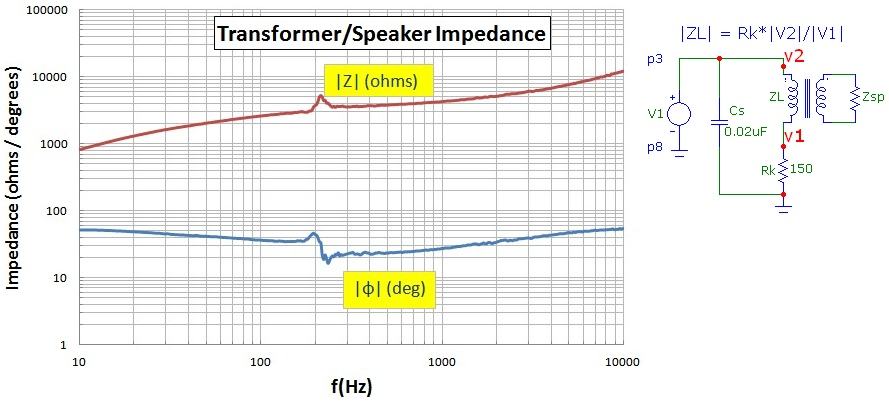## Reference

The schematic diagram of a generic AA5 radio (RCA Receiving Tube Manual, 1961 p.448) similar to the Mantola radio discussed above is shown below. The tubes used in the Mantola R654-PM are shown also. The Mantola set uses an CLC power supply filter instead of the simple C16 filter below. Of course the component values shown below will be different for the Mantola radio: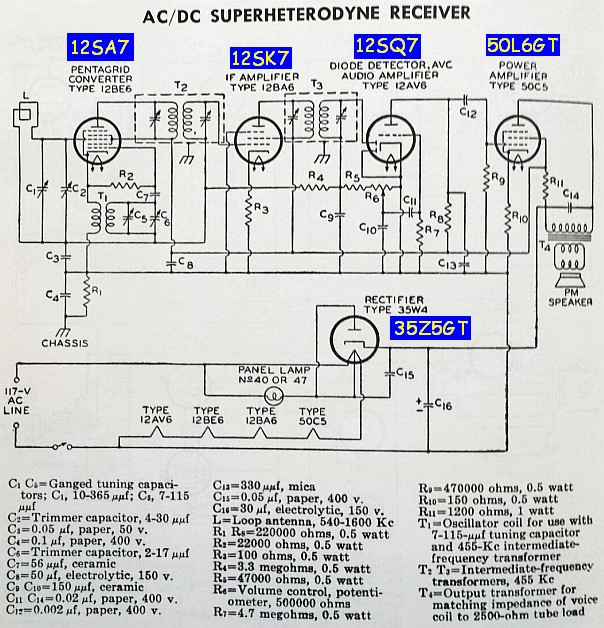References